Courses

# Study Notes for Flywheel Notes | EduRev

## Mechanical Engineering : Study Notes for Flywheel Notes | EduRev

The document Study Notes for Flywheel Notes | EduRev is a part of the Mechanical Engineering Course Theory of Machines (TOM).
All you need of Mechanical Engineering at this link: Mechanical Engineering

Introduction

• If the components of a machine accelerate, inertia forces are produced due to their masses. However, if the magnitudes of these forces are small compared to the externally applied loads, they can be neglected while analysing the mechanism. Such an analysis is known as static-force analysis.
• For example, in lifting cranes, the bucker load and the static weight loads may be quite high relative to any dynamic loads due to accelerating masses, and thus static-force analysis is justified.
• When the inertia effect due to the mass of the components is also considered, it is called dynamic-force analysis.

Kinematic Analysis of Single-Slider Crank Mechanism

• Figure below shows a slider-crank mechanism in which the crank OA rotates in the clockwise direction. ℓ and r are the lengths of the connecting rod and the crank respectively.
• In kinematic analysis, inertia of connecting rod is not considered in this analysis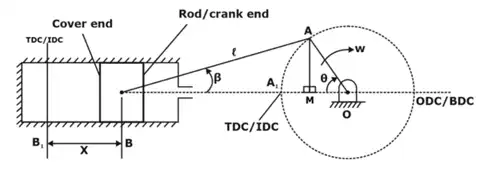Schematic of single-slider crank mechanism
ω = dθ/dt
sinβ = 1/n sinθ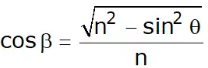1. Displacement Analysis (x) of piston
Let x = displacement of piston from inner-dead centre at the moment when the crank has turned through angle θ from the inner-dead centre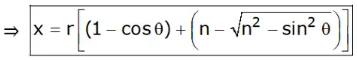2. Velocity Analysis (V) of piston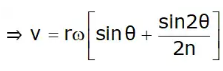3. Acceleration Analysis (f) of piston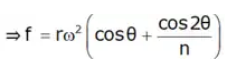← Acceleration of Reciprocating mass
4. Angular Velocity of Connecting rod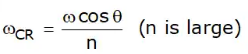5. Angular Acceleration of Connecting rod
Let ∝CR = angular acceleration of the connecting Rod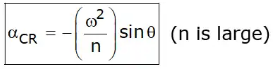Dynamic-Analysis of Single-Slider Crank Mechanism

An engine is acted upon by various forces such as weight of reciprocating masses and connecting rod, gas forces, forces due to friction and inertia due to acceleration and retardation of engine elements, the last being dynamic in nature.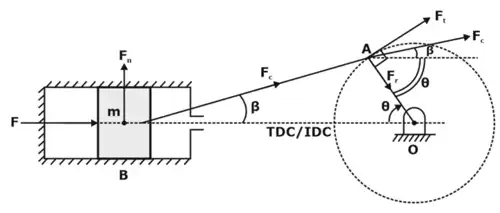Fig.2: Dynamics of single-slider crank mechanism

Total Piston Effort (F)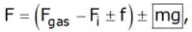(mg is used for vertical engines)
Force/thrust along connecting Rod (Fc)
Let Fc = Force In the connecting rod (Fig. 2)
Then equating the horizontal components of forces,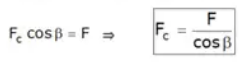Normal thrust to cylinder walls (Fn)
It Is the normal reaction on the cylinder walls.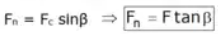Radial thrust on crank shaft Bearings (Fr
The component of FC along the crank (In the radial direction) produces a thrust on the crankshaft bearings.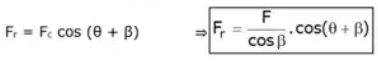Crank Effort (Ft)
Force Is exerted on the crankpln as a result of the force on the piston. Crank effort Is the net effort (force) applied at the crankpln perpendicular to the crank which gives the required turning moment on the crankshaft. It Is the force needed to drive crank.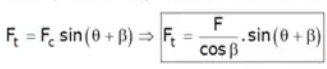Turning moment on crank-Shaft: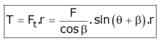Flywheel

• A flywheel acts as an energy reservoir, which stores energy during the period when the supply of energy is more than the requirement and releases energy during the period when the requirement is more than the supply.
• In case a variable torque is supplied where demand is a constant torque or demand is variable torque whereas constant torque is supplied. In both these cases there is mismatch between the supply and demand. This results in speed variation.
1. Fluctuation of Energy
(i) The fluctuation of energy may be determined by the turning moment diagram for one complete cycle of operation. The variations of energy above and below the mean resisting torque line are called fluctuations of energy.
(ii) The difference between the greatest speed and the least speed is known as the maximum fluctuation of speed and the ratio of the maximum fluctuation of speed to mean speed is define as the coefficient of fluctuation of speed.
Maximum fluctuation of speed = Nmax -Nmin
Coefficent of fluctuation of Speed, Cs = (Nmax -Nmin)/(Nmean)
(Nmean = mean speed of engine)
(iii) The difference between the maximum and minimum kinetic engines of the flywheel is known as the maximum fluctuation of energy.
ΔE = Iω2Cs
2. Requirement of Flywheel in Power Presses
(i) When the load on the crankshaft is constant or varies and the input torque varies continuously during a cycle, a flywheel is used to reduce the fluctuations of speed.
(ii) A flywheel can perform the same purpose in a punching press or a riveting machine in which the torque available is constant but the load varies during the cycle.
3. Designing of Flywheel Rim
Below we are studying Rim (Ring shaped) type flywheel, which is practical not usable.
Let, A → cross section area of the rim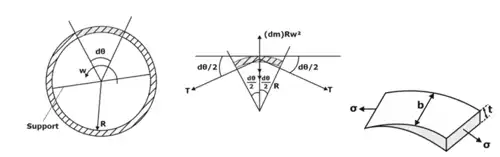Fig.3: Rim type Flywheel
Fig.4: Cross-section of flywheel
For safe designing, σ ≤ σb (bearing strength)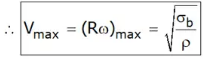Offer running on EduRev: Apply code STAYHOME200 to get INR 200 off on our premium plan EduRev Infinity!

## Theory of Machines (TOM)

94 videos|41 docs|28 tests

,

,

,

,

,

,

,

,

,

,

,

,

,

,

,

,

,

,

,

,

,

;